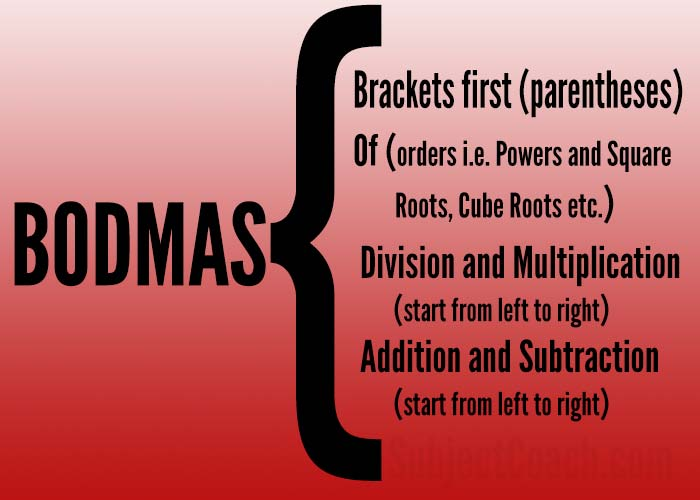# What is BODMAS

### What is BODMAS and how can it be used to simplify operator precedence

BODMAS as a term means

Brackets First of Operations/Orders/Exponents (Powers, Square Roots, Cube Roots etc) before Multiply, Divide, Add or Subtract

then

Multiply or Divide before you Add or Subtract

after which you perform

Here is one example

7 × (4 + 2) = 7 × 6   = 42 is Correct7 × (4 + 2) = 28 + 2 = 30  is Wront

Here is a picture that you can print and keep it for your reference## Things to keep in mind

1. Start Divide/Multiply from left side to right side since they perform equally.
2. Start Add/Subtract from left side to right side since they perform equally.

Let's try to understand this by taking few examples

### Brackets First

Example 1

7 × (4 + 2) = 7 × 6 = 42 is Correct 

Example 2

(3+3)*(15-5)*10 =6*10*10 = 600is correct

### Exponents

Example 1

2 × 42 + (1+3) = 2 × 42 + 4 = 2 x 16 + 4= 32 + 4 =36

Example 2

(3 + 2)2 =52 =10

Multiply or divide (go left to right) before you perform addition or Subtraction

Example 1

30/15-10 = 2 - 10 = -8

Example 2

30* 2 + (15-10) = 30 * 2 + 5 = 60 + 5 = 65

### Addition and Subtraction (go left to right)

Example 1

30 + 15 - 10 = 45 - 10 = 35

Example 2

30 - 15 + 10 = 15 + 10 = 25 

Let's take a complex equation as shown below

(20 - 4 * 4) + (2 × 32 + 6) = (Start inside brackets and start solving by operator precedence)(20 - 16) + (2 x 9 + 6) = (solve multiply in first brackets and exponent in second one)4 + (18 + 6) = (solve first bracket and perform multiply before addition)4 + 24 = (solve second bracket)28 (add all)

So you see how easy it becomes with BODMAS. Try solving Quiz questions and see how you perform. Check out other interesting Maths tutorials on SubjectCoach to get you started on various topics.

### Description

This is a quick tip tutorial for you to understand what BODMAS is and how we can utilize it to make calculations simple.

This tutorial will be followed up by a Quiz

### Prerequisites

Deep understanding of Number including Addition, Multiplication, Division, Subtraction etc

### Audience

Students new to BODMAS

### Learning Objectives

We are going to learn what BODMAS means and how we can simplify order of operations using BODMAS

Author: Subject Coach
You must be logged in as Student to ask a Question.